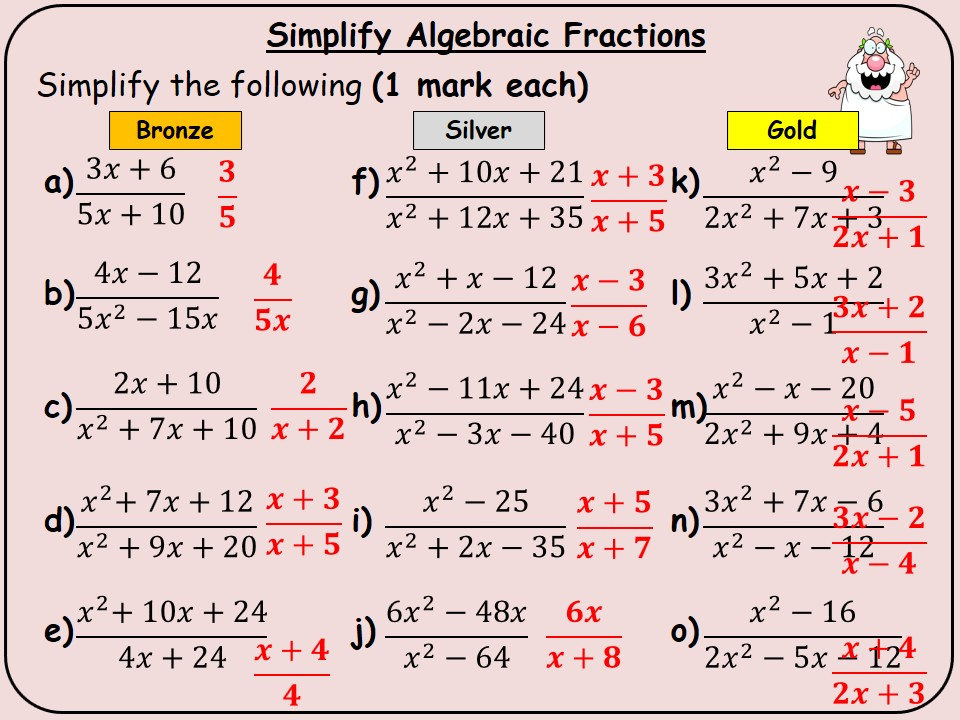I tend to create worksheets or homework's aimed at GCSE students.

Last updated

11 November 2018

#### Share thispptx, 1.89 MB

A powerpoint presentation that contains 50 differentiated sets of questions (Bronze/ Silver/ Gold).

Generally there are 2 or 3 levels of differentiation (sometimes just silver and gold, other slides contain gold, silver and bronze)

It contains a contents page with hyperlinked topics for easy access to questions.

There are a total of 50 seperate topics included on this Powerpoint. Answers are animated and appear on a click. Each slide is approximately 10 - 20 minutes worth of work - depending on the topic.

I have also left at the end of the slide starters i have created within the powerpoint. It is fairly easy to copy and paste questions and answers to create seperate questions to project.

The full list includes

Algebra
Expand double
Expand two single
Expand one bracket
list integers from inequalities
Collect like terms
Factorise double
Nth term - linear
Factorise single
Interpret sequences
Generate linear
Factorise double and single
Solving equations both sides - integer solutions
Finding a term in a sequence
Simultaneous equations - Positive answers
Quadratic sequences
Linear equations - with brackets
linear equations - with fractions
solve quadratcis by factorising
factorise double brackets (non 1 coef of x squared)
Solve inequalities
Expand triple brackets
Quadratic formula
Rearrange the formula
Simplify algebraic fractions
Foctorise do2s
Rearange the formula - 2

**Number **

Compound interest
Depreciation
Reverse percentages
Recurring Decimals
Decimal Multiplication
Reverse compound interest
Add subtract fractions
Indices - negative and fractional
Simple interest
Add and subtract standard form
Estimatation
Multiply and divide standard form
Median, LQ, UQ
Error intervals
Convert standard form

Geometry
Pyhtagoras
Mixed Sin and Cos rule
Sin Rule
Cosine Rule
0.5abSinC
Area and circunference of circles
SOHCAHTOA - Mixed
Area and perimeter of semi circles

### Review

5

#### pmasong2

2 years ago
5

Report this resourceto let us know if it violates our terms and conditions.
Our customer service team will review your report and will be in touch.

£10.00